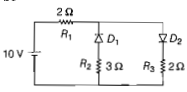# NEET Physics Semiconductor Electronics Questions SolvedNEET - 2016

The given circuit has two ideal diodes connected as shown in the figure below. The current flowing through the resistance ${R}_{1}$ will be(a) 2.5 A                     (b) 10.0 A

(c) 1.43 A                   (d) 3. 13 A

(a) We know that a diode only conducts in forward biased condition. In the given circuit. the diode ${D}_{1}$ will be in reverse bias, so it will block the current and diode ${D}_{2}$ will be in forward bias, so it will pass the current

$i=\frac{v}{{R}_{1}+{R}_{2}}=\frac{10}{2+2}=2.5A$

Difficulty Level:

• 61%
• 18%
• 14%
• 8%
Crack NEET with Online Course - Free Trial (Offer Valid Till September 21, 2019)